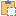An object that represents a force/moment applied in a linear manner.

Namespace: Autodesk.Revit.DB.Structure
Assembly: RevitAPI (in RevitAPI.dll) Version: 21.0.0.0 (21.1.1.109)

# Syntax

C#
``public class LineLoad : LoadBase``
Visual Basic
``````Public Class LineLoad _
Visual C++
``public ref class LineLoad : public LoadBase``

# Remarks

The load/moment may be uniform or it may vary between the two ends of the line.

# ExamplesCopy C#
`````` private void LineLoadInformation(LineLoad lineLoad)
{
StringBuilder information = new StringBuilder("Line Load: ");

// Get the load case name

//Get the three dimensional force applied to the start point and end point of the line load.

//Get the three dimensional location of the start point and end point for the line load.

//Get the three dimensional moment applied to the start point and end point of the line load.

//Whether the load is projected or not
{
}
else
{
information.Append("\nThis line load is not projected");
}

//Report if the load is uniform
{
}
else
{
}

//show information of lineload in a MessageBox.
}

// output the point's three coordinates
string XYZToString(XYZ point)
{
return "(" + point.X + ", " + point.Y + ", " + point.Z + ")";
}``````Copy VB.NET
``````Private Sub LineLoadInformation(lineLoad As LineLoad)
Dim information As New StringBuilder("Line Load: ")

' Get the load case name

'Get the three dimensional force applied to the start point and end point of the line load.
information.Append(vbLf & "Force at the start of this line load: " & XYZToString(lineLoad.ForceVector1))
information.Append(vbLf & "Force at the end of this line load: " & XYZToString(lineLoad.ForceVector2))

'Get the three dimensional location of the start point and end point for the line load.

'Get the three dimensional moment applied to the start point and end point of the line load.
information.Append(vbLf & "Moment at the start of this line load: " & XYZToString(lineLoad.MomentVector1))
information.Append(vbLf & "Moment at the end of this line load: " & XYZToString(lineLoad.MomentVector2))

'Whether the load is projected or not
information.Append(vbLf & "This line load is projected.")
Else
information.Append(vbLf & "This line load is not projected")
End If

'Report if the load is uniform
information.Append(vbLf & "This load is uniform.")
Else
information.Append(vbLf & "This load is not uniform.")
End If

'show information of lineload in a MessageBox.
End Sub

' output the point's three coordinates
Private Function XYZToString(point As XYZ) As String
Return "(" & Convert.ToString(point.X) & ", " & Convert.ToString(point.Y) & ", " & Convert.ToString(point.Z) & ")"
End Function``````

# Inheritance Hierarchy

System Object
Autodesk.Revit.DB Element